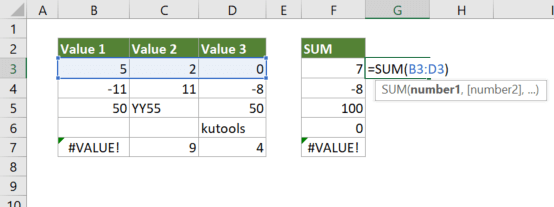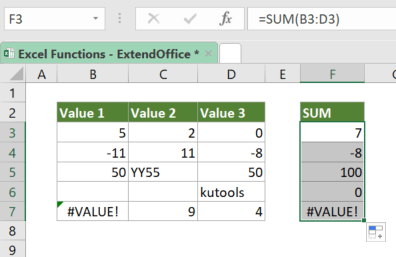## Excel SUM Function

The SUM function adds all values you specified. The values can be numbers, cell references, ranges, arrays or a mix of all four.#### Syntax

=SUM(number1, [number2], ...)

#### Arguments

• number1 (required): The first value to be summed.
• [number2], … (optional): The second and up to 253 more values to be summed.

#### Return Value

The SUM function returns a numeric value.

#### Function Notes

• If there are any error values in any of the arguments, SUM will return the first error value.
• SUM treats blank cells as 0s.
• If a text value that cannot be interpreted as a numeric value is referenced by the SUM function, SUM will treat it as 0; If the text value is supplied directly to the SUM (e.g., =SUM(“kutools”)), the function will return the #VALUE! error.
• If a logical value is referenced by the SUM function, SUM will treat it as 0; If the logical value is supplied directly to the SUM, the function will treat is as a number (TRUE=1, FALSE=0).

#### Example

To get the sum of the numbers on the first row of the table as shown below, please copy or enter the formula below in cell F3, and press Enter to get the result:

=SUM(5,2,0)

√ Note: In the formula above, we used the actual values. However, in the case that you have a table as shown below, cell references are better choices than the actual values. Since you can just drag the fill handle down to apply the formula to the below cells after entering the formula with cell references in the top cell. The formula with cell references is as followed:

=SUM(B3:D3)#### Related functions

Excel SUMIF Function

In Excel, the SUMIF function can help to sum cells based on one criterion, this article will explain this function's syntax and introduce some examples for using the SUMIF function.

Excel SUMIFS Function

The SUMIFS function in Excel can help to sum cell values based on multiple criteria, this tutorial will explain the SUMIFS function's syntax and some examples for using this function.

Excel SUMPRODUCT Function

In Excel, the SUMPRODUCT function can be used to multiply two or more columns or arrays together, and then get the sum of products. In fact, the SUMPRODUCT is a useful function which can help to count or sum cell values with multiple criteria like the COUNTIFS or SUMIFS function. This article will introduce the function syntax and some examples for this SUMPRODUCT function.

Excel SUMSQ Function

The SUMSQ function returns the sum of squares of supplied values.

### The Best Office Productivity Tools

#### Kutools for Excel - Helps You To Stand Out From Crowd

 Popular Features: Find, Highlight or Identify Duplicates  |  Delete Blank Rows  |  Combine Columns or Cells without Losing Data  |  Round without Formula ... Super VLookup: Multiple Criteria  |  Multiple Value  |  Across Multi-Sheets  |  Fuzzy Lookup... Adv. Drop-down List: Easy Drop Down List  |  Dependent Drop Down List  |  Multi-select Drop Down List... Column Manager: Add a Specific Number of Columns  |  Move Columns  |  Toggle Visibility Status of Hidden Columns  |  Compare Columns to Select Same & Different Cells ... Featured Features: Grid Focus  |  Design View  |  Big Formula Bar  |  Workbook & Sheet Manager | Resource Library (Auto Text)  |  Date Picker  |  Combine Worksheets  |  Encrypt/Decrypt Cells  |  Send Emails by List  |  Super Filter  |  Special Filter (filter bold/italic/strikethrough...) ... Top 15 Toolsets:  12 Text Tools (Add Text, Remove Characters ...)  |  50+ Chart Types (Gantt Chart ...)  |  40+ Practical Formulas (Calculate age based on birthday ...)  |  19 Insertion Tools (Insert QR Code, Insert Picture from Path ...)  |  12 Conversion Tools (Numbers to Words, Currency Conversion ...)  |  7 Merge & Split Tools (Advanced Combine Rows, Split Excel Cells ...)  |  ... and more

Kutools for Excel Boasts Over 300 Features, Ensuring That What You Need is Just A Click Away...#### Office Tab - Enable Tabbed Reading and Editing in Microsoft Office (include Excel)

• One second to switch between dozens of open documents!
• Reduce hundreds of mouse clicks for you every day, say goodbye to mouse hand.
• Increases your productivity by 50% when viewing and editing multiple documents.
• Brings Efficient Tabs to Office (include Excel), Just Like Chrome, Edge and Firefox.